## 机器翻译：谷歌翻译是如何对几乎所有语言进行翻译的？

收藏

分享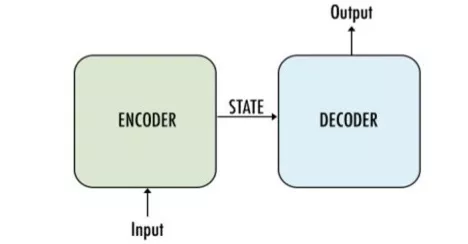· 编码器：使用多个深度神经网络层，将输入单词转换为相应的隐藏向量。每个向量代表当前单词及其语境。

· 解码器：与编码器类似。它将编码器生成的隐藏向量、自身的隐藏状态和当前单词作为输入，从而生成下一个隐藏向量，最终预测下一个单词。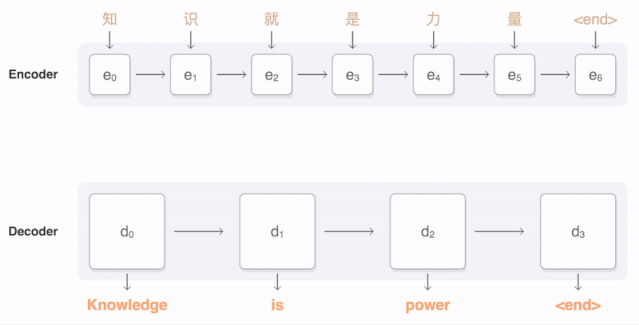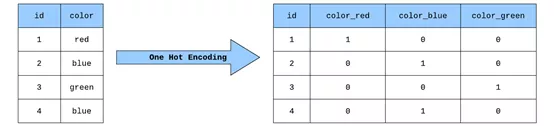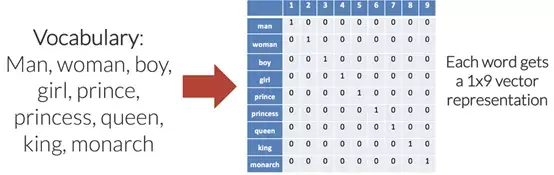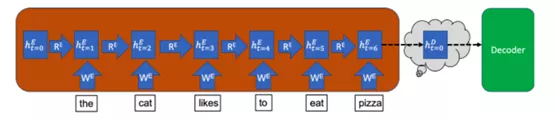1. #Import Libraries
2.          import os, sys
3.          from keras.models importModel
4.          from keras.layers importInput, LSTM, GRU, Dense, Embedding
5.          fromkeras.preprocessing.text importTokenizer         fromkeras.preprocessing.sequence import pad_sequences
6.          from keras.utils import to_categorical
7.          import numpy as np
8.          import pandas as pd
9.          import pickle
10.          importmatplotlib.pyplot as plt
11.              #Values fordifferent parameters:         BATCH_SIZE=64
12.          EPOCHS=20
13.          LSTM_NODES=256
14.          NUM_SENTENCES=20000
15.          MAX_SENTENCE_LENGTH=50
16.          MAX_NUM_WORDS=20000
17.          EMBEDDING_SIZE=200

1. input_sentences = []
2.                 output_sentences = []                output_sentences_inputs = []             count =0
3.                 for line inopen('./drive/MyDrive/fra.txt', encoding="utf-8"):
4.                     count +=1
5.                     if count >NUM_SENTENCES:
6.                         break
7.                     if'\t'notin line:
8.                         continue
9.                     input_sentence = line.rstrip().split('\t')
10.                     output = line.rstrip().split('\t')
11.                  output_sentence = output +' <eos>'
12.                     output_sentence_input ='<sos> '+ output
13.                  input_sentences.append(input_sentence)
14.                     output_sentences.append(output_sentence)
15.                     output_sentences_inputs.append(output_sentence_input)
16.              print("Number ofsample input:", len(input_sentences))
17.                 print("Number ofsample output:", len(output_sentences))
18.                 print("Number ofsample output input:", len(output_sentences_inputs))
` `
1. Output:
2. Number of sample input: 20000
3. Number of sample output: 20000
4. Number of sample output input: 20000

1. print("English sentence: ",input_sentences)
2. print("French translation: ",output_sentences)
3. Output:English sentence:  Join us.French translation:  Joignez-vous à nous.<eos>

1. eng_len = []
2.        fren_len = []             # populate thelists with sentence lengths       for i ininput_sentences:
3.             eng_len.append(len(i.split()))
4.              for i inoutput_sentences:
5.             fren_len.append(len(i.split()))
6.              length_df = pd.DataFrame({'english':eng_len, 'french':fren_len})
7.              length_df.hist(bins =20)
8.        plt.show()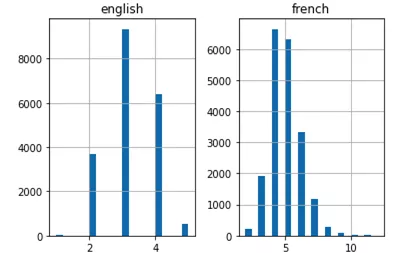1. #tokenize the input sentences(inputlanguage)
2.                 input_tokenizer =Tokenizer(num_words=MAX_NUM_WORDS)                input_tokenizer.fit_on_texts(input_sentences)                input_integer_seq = input_tokenizer.texts_to_sequences(input_sentences)                print(input_integer_seq)
3.              word2idx_inputs =input_tokenizer.word_index                print('Total uniquewords in the input: %s'%len(word2idx_inputs))
4.              max_input_len =max(len(sen) for sen in input_integer_seq)
5.                 print("Length oflongest sentence in input: %g"% max_input_len)
` `
1. Output:
2. Total unique words in the input: 3501
3. Length of longest sentence in input: 6

1. #tokenize theoutput sentences(Output language)
2. output_tokenizer =Tokenizer(num_words=MAX_NUM_WORDS, filters='')
3. output_tokenizer.fit_on_texts(output_sentences+output_sentences_inputs)  output_integer_seq =output_tokenizer.texts_to_sequences(output_sentences)  output_input_integer_seq =output_tokenizer.texts_to_sequences(output_sentences_inputs)  print(output_input_integer_seq)
4.            word2idx_outputs=output_tokenizer.word_index  print('Total uniquewords in the output: %s'%len(word2idx_outputs))
5.            num_words_output=len(word2idx_outputs)+1
6. max_out_len =max(len(sen) for sen inoutput_integer_seq)
7. print("Length oflongest sentence in the output: %g"% max_out_len)
` `
1. Output:
2. Total unique words in the output: 9511
3. Length of longest sentence in the output: 12

4.  print("decoder_input_sequences.shape:",decoder_input_sequences.shape)
6.  print("decoder_output_sequences.shape:",decoder_output_sequences.shape)
` `
1. encoder_input_sequences.shape: (20000, 6)
2. decoder_input_sequences.shape: (20000, 12)
3. decoder_output_sequences.shape: (20000, 12)

1. print("encoder_input_sequences:",encoder_input_sequences)Output:
2. encoder_input_sequences: [  0   0  0   0 464  59]

1. prnt(word2idx_inputs["join"])
2. print(word2idx_inputs["us"])Output:
3. 464
4. 591. from numpy import array
2.  from numpy import asarray
3.  from numpy import zeros
4.             embeddings_dictionary=dict()             glove_file =open(r'./drive/My Drive/glove.twitter.27B.200d.txt', encoding="utf8")
5.             for line in glove_file:
6.      rec = line.split()      word= rec
7.     vector_dimensions =asarray(rec[1:], dtype='float32')
8.     embeddings_dictionary[word] = vector_dimensions  glove_file.close()

1. num_words =min(MAX_NUM_WORDS, len(word2idx_inputs)+1)
2.                      embedding_matrix =zeros((num_words, EMBEDDING_SIZE))                     for word, index inword2idx_inputs.items():                         embedding_vector = embeddings_dictionary.get(word)                         if embedding_vector isnotNone:                             embedding_matrix[index] =embedding_vector

· input_dim：表示文本数据中词汇表的容量。比如，如果数据被整数编码为0-10之间的值，那么词汇表的容量为11个单词。

· output_dim：表示将嵌入单词的向量空间大小。它决定该层每个单词的输出向量大小。比如，它可以是32或100，甚至还可以更大。如果大家对此有疑问，可以用不同的值测试。

· input_length：表示输入序列的长度，正如大家为Keras模型的输入层所定义的那样。比如，如果所有的输入文档都由1000个单词组成，那么该值也为1000。

1. embedding_layer = Embedding(num_words, EMBEDDING_SIZE,weights=[embedding_matrix], input_length=max_input_len)

1. #shape of the output
2. decoder_targets_one_hot = np.zeros((len(input_sentences), max_out_len,num_words_output),
3. dtype='float32'
4. )decoder_targets_one_hot.shapeShape: (20000, 12, 9512)

1. for i, d in enumerate(decoder_output_sequences):
2.     for t, word in enumerate(d):
3.         decoder_targets_one_hot[i, t,word] = 1

1. encoder_inputs =Input(shape=(max_input_len,))
2.                               x =embedding_layer(encoder_inputs)                              encoder =LSTM(LSTM_NODES, return_state=True)
3.              encoder_outputs,h, c =encoder(x)                              encoder_states = [h, c]

1. decoder_inputs =Input(shape=(max_out_len,))
2. decoder_embedding =Embedding(num_words_output,LSTM_NODES)  decoder_inputs_x =decoder_embedding(decoder_inputs)             decoder_lstm =LSTM(LSTM_NODES,return_sequences=True, return_state=True)
3. decoder_outputs, _, _ =decoder_lstm(decoder_inputs_x,initial_state=encoder_states)             #Finally, theoutput from the decoder LSTM is passed through a dense layer to predict decoderoutputs.
4. decoder_dense =Dense(num_words_output,activation='softmax')
5. decoder_outputs =decoder_dense(decoder_outputs)

1. #Compile
2.         model =Model([encoder_inputs,decoder_inputs],decoder_outputs)
3.         model.compile(
4.            optimizer='rmsprop',
5.            loss='categorical_crossentropy',
6.            metrics=['accuracy']
7.         )
8.         model.summary()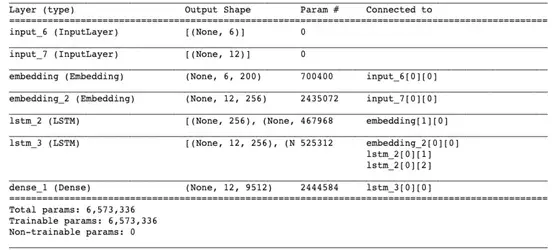1. es =EarlyStopping(monitor='val_loss', mode='min', verbose=1)
2.              history = model.fit([encoder_input_sequences,decoder_input_sequences], decoder_targets_one_hot,                                      batch_size=BATCH_SIZE,                                      epochs=20,
3.                                       callbacks=[es],                                      validation_split=0.1,
4.                                   )

1. model.save('seq2seq_eng-fra.h5')

1. #Accuracy
2. plt.title('model accuracy')
3. plt.plot(history.history['accuracy'])
4. plt.plot(history.history['val_accuracy'])
5. plt.ylabel('accuracy')
6. plt.xlabel('epoch')
7. plt.legend(['train', 'test'], loc='upper left')
8. plt.show()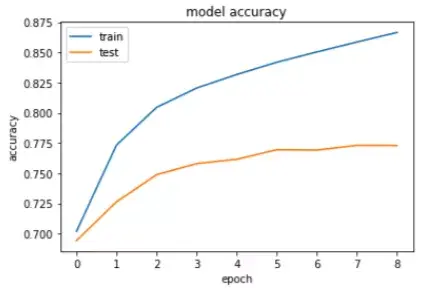1. encoder_model = Model(encoder_inputs, encoder_states)
2. model.compile(optimizer='rmsprop', loss='categorical_crossentropy')

· 编码输入序列，返回其内部状态。

· 仅使用start-of-sequence字符作为输入，并使用编码器内部状态作为解码器的初始状态来运行解码器。

· 将解码器预测的字符（在查找令牌之后）添加到解码序列中。

· 将先前预测的字符令牌作为输入，重复该过程，更新内部状态。

1. decoder_state_input_h =Input(shape=(LSTM_NODES,))
2.                            decoder_state_input_c=Input(shape=(LSTM_NODES,))                           decoder_states_inputs=[decoder_state_input_h, decoder_state_input_c]             decoder_inputs_single=Input(shape=(1,))
3.                            decoder_inputs_single_x=decoder_embedding(decoder_inputs_single)             decoder_outputs,h, c =decoder_lstm(decoder_inputs_single_x, initial_state=decoder_states_inputs)             decoder_states = [h, c]                           decoder_outputs =decoder_dense(decoder_outputs)             decoder_model =Model(                               [decoder_inputs_single] +decoder_states_inputs,                               [decoder_outputs] + decoder_states

1. idx2word_input = {v:k for k, v inword2idx_inputs.items()}
2. idx2word_target = {v:k for k, v inword2idx_outputs.items()}

1. deftranslate_sentence(input_seq):
2.                                    states_value = encoder_model.predict(input_seq)                                   target_seq = np.zeros((1, 1))
3.                                    target_seq[0, 0] =word2idx_outputs['<sos>']
4.                                    eos = word2idx_outputs['<eos>']
5.                                    output_sentence = []                 for _ inrange(max_out_len):
6.                                        output_tokens, h, c = decoder_model.predict([target_seq] + states_value)                                       idx = np.argmax(output_tokens[0, 0, :])
7.                      if eos == idx:
8.                                            break
9.                      word =''
10.                      if idx >0:
11.                                            word =idx2word_target[idx]                                           output_sentence.append(word)                     target_seq[0, 0] = idx
12.                                        states_value = [h, c]                 return' '.join(output_sentence)

1. i = np.random.choice(len(input_sentences))
2.                                        input_seq=encoder_input_sequences[i:i+1]
3.                                        translation=translate_sentence(input_seq)                                       print('Input Language: ', input_sentences[i])
4.                                        print('Actualtranslation : ', output_sentences[i])
5.                                        print('Frenchtranslation : ', translation)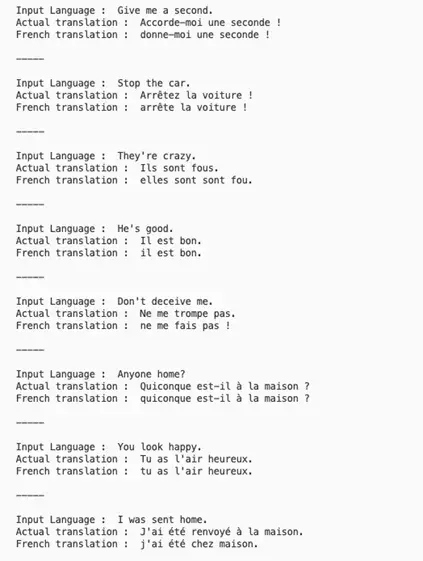【编辑推荐】

0

#### 相关文章推荐